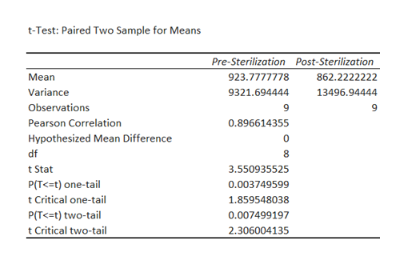# Factor V is a protein involved in the forming of blood clots. The higher the level of factor V, the faster the blood clots. A Blood Transfusions Service is interested in the effects of sterilization of blood plasma because factor V is unstable and may break down during sterilization. The table below gives measured levels of factor V in blood samples from nine donors. Both pre- and post-sterilization measurements are given for each person. Test whether the mean factor V levels are different post-sterilization. Run the test at a 5% level of significance. Give each of the following  1) the appropriate null and alternative hypotheses; 2) the appropriate test; 3) the decision rule; 4) the calculation of the test statistic; and 5) your conclusion including a comparison to alpha or the critical value. Factor V measurementsDonor numberPre-SterilizationPost-Sterilization110259852106010303953923484789257506506855759794782889788849899809

Question
44 views

Factor V is a protein involved in the forming of blood clots. The higher the level of factor V, the faster the blood clots. A Blood Transfusions Service is interested in the effects of sterilization of blood plasma because factor V is unstable and may break down during sterilization. The table below gives measured levels of factor V in blood samples from nine donors. Both pre- and post-sterilization measurements are given for each person. Test whether the mean factor V levels are different post-sterilization. Run the test at a 5% level of significance. Give each of the following  1) the appropriate null and alternative hypotheses; 2) the appropriate test; 3) the decision rule; 4) the calculation of the test statistic; and 5) your conclusion including a comparison to alpha or the critical value.

Factor V measurements

 Donor number Pre-Sterilization Post-Sterilization 1 1025 985 2 1060 1030 3 953 923 4 847 892 5 750 650 6 855 759 7 947 828 8 978 884 9 899 809
check_circle

Step 1

(1)

Here, the claim of the test is that the mean factor V levels are different post-sterilization.

The test hypotheses are given below:

Step 2

(2)

In matched-pair design, the subjects are paired according to particular characteristics after which a subject is assigned to a treatment group and another subject.

Here, the samples are dependent. Hence, paired t test is appropriate.

(3)

Rejection rule:

Step 3

(4)

Step-by-step procedure to obtain the test statistic and p-value using Excel:

• In Excel sheet, enter Pre-Sterilization in one column and enter Post-Sterilization in one column.
• In Data, select Data Analysis and choose t-test; Paired Two Samples for Means.
• In Input Variable 1 Range, select Pre-Sterilization.
• In Input Variable 2 Range, select Post-Sterilization.
• In Hypothesized Mean D...help_outlineImage Transcriptioncloset-Test: Paired Two Sample for Means Pre-Sterilization Post-Sterilization 862.2222222 Мean 923.7777778 Variance 9321.694444 13496.94444 Observations 9 Pearson Correlation 0.896614355 Hypothesized Mean Difference df 8 tStat 3.550935525 P(T<=t) one-tail 0.003749599 tCritical one-tail 1.859548038 P(T

### Want to see the full answer?

See Solution

#### Want to see this answer and more?

Solutions are written by subject experts who are available 24/7. Questions are typically answered within 1 hour.*

See Solution
*Response times may vary by subject and question.
Tagged in

### Hypothesis Testing